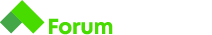0

Здравейте,

Имам проблем със следната задача:

# Converter

You want to buy this really cool car from the UK, but you are worried about the fuel consumption. The values you see are MPG (miles per gallon). You have no idea what 20 MPG means so, being a programmer, decide to write a converter that helps you calculate the consumption.

Doing some research, you learn that `1 gallon = 4.54 litres` and `1 mile = 1.6 km` .

After the calculation, round the result down to the neareast whole number.

## Input

• On the first line you will receive a number m - miles per galon

## Output

• On the only line of output, print `{result} litres per 100 km`

## Constraints

• 1 <= m <= 100

## Input

``````20
``````

## Output

``````14 litres per 100 km
``````

Ето го и кода ми:
import java.util.Scanner;

public class Main {
public static void main(String[] args) {
Scanner input = new Scanner(System.in);
Integer m = input.nextInt();;
double mile = 1.6;
double gallon = 4.54;
double kmp = Math.floor((100gallon)/(milem));
System.out.println(kmp + " liter per 100 km");

``````}
``````

}

Здравей,

пробвай, ако промениш “Integer m = input.nextInt();;” с “int m = Integer.parseInt(input.nextLine());”
Ето го моя код:
int m = Integer.parseInt(scan.nextLine());
double resultLitres = Math.floor(((100 / 1.6) / m) * 4.54);
System.out.printf("%.0f" + " litres per 100 km", resultLitres);

Успех!Здравей,

Сега работи пефеткно! Благодаря ти за помоща!